# Can not able to load inputs and labels to GPU

firstly i added data generators for train and val

now when i am iterating over the dataloader from the dictionary created on gpu it is giving me the following error :–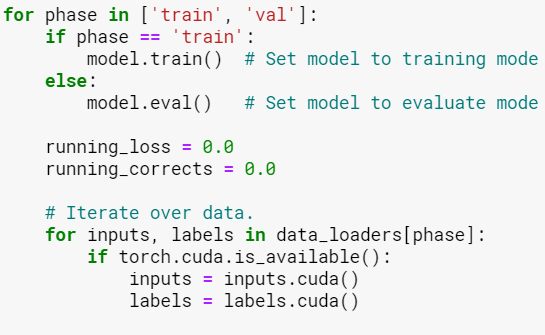Please don’t tag certain persons, as this might discourage others to answer.
Also, it’s not a good idea to tag all admins when creating the topic.

That being said, it seems that your `input` is a `str` object while a tensor is expected.
I would recommend to check the implementation of your `Dataset` and make sure that tensors are returned.

This was the Dataset which I implemented

then i did the sanity check

which returned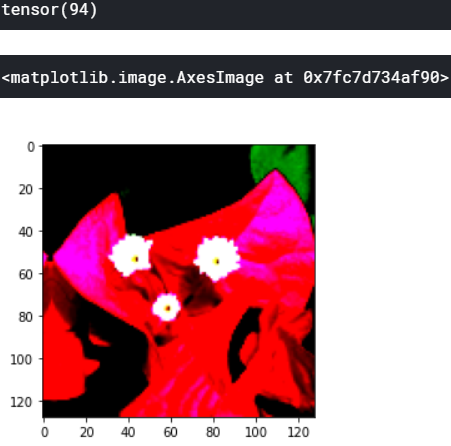and then I did

among which the train dataloder is working fine while the validation dataloader not.
I did the same exact steps after the above code mentioned in the following topic

I would strongly suggest you not to put images but real code and possible a small reprudicible code snippet so we can try it outYou will get much more people to answer

so this was the dataset class which i created

# defining dataset

``````from PIL import Image
import cv2
import albumentations
import torch
import numpy as np
import io
from torch.utils.data import Dataset

class FlowerDataset(Dataset):
def __init__(self, id , classes , image , img_height , img_width, mean , std , is_valid):
self.id = id
self.classes = classes
self.image = image
if is_valid == 1:
self.aug = albumentations.Compose([
albumentations.Resize(img_height , img_width, always_apply = True) ,
albumentations.Normalize(mean , std , always_apply = True)
])
else:
self.aug = albumentations.Compose([
albumentations.Resize(img_height , img_width, always_apply = True) ,
albumentations.Normalize(mean , std , always_apply = True),
albumentations.ShiftScaleRotate(shift_limit = 0.0625,
scale_limit = 0.1 ,
rotate_limit = 5,
p = 0.9)
])

def __len__(self):
return len(self.id)

def __getitem__(self, index):
id = self.id[index]
img = np.array(Image.open(io.BytesIO(self.image[index])))
img = cv2.resize(img, dsize=(128, 128), interpolation=cv2.INTER_CUBIC)
img = self.aug(image = img)['image']
img = np.transpose(img , (2,0,1)).astype(np.float32)

return {
'image' : torch.tensor(img, dtype = torch.float),
'class' : torch.tensor(self.classes[index], dtype = torch.long)
}
``````

and after that I did the sanity check which also worked fine

# sanity check for FlowerDataset class created

``````train_dataset = FlowerDataset(id = train_ids, classes = train_class, image = train_images,
img_height = 128 , img_width = 128,
mean = (0.485, 0.456, 0.406),
std = (0.229, 0.224, 0.225) , is_valid = 0)

val_dataset = FlowerDataset(id = val_ids, classes = val_class, image = val_images,
img_height = 128 , img_width = 128,
mean = (0.485, 0.456, 0.406),
std = (0.229, 0.224, 0.225) , is_valid = 1)

import matplotlib.pyplot as plt
%matplotlib inline

idx = 119
img = val_dataset[idx]['image']

print(val_dataset[idx]['class'])

npimg = img.numpy()
plt.imshow(np.transpose(npimg, (1,2,0)))
``````

The code looks generally alright.
I would suggest to loop once over the complete dataset and check, if the returned batches are indeed tensors or if at some index a `str` object might be returned.
If this works, then you should check, if you are reassigning `input` somewhere in your code to a `str`.

When I loop over the train datalader as -->

# setting up the train data loader

``````!pip install git+https://github.com/ildoonet/cutmix
from cutmix.cutmix import CutMix

num_class=104,
beta=1.0,
prob=0.5,
num_mix=2)

if torch.cuda.is_available():
inputs = inputs.cuda()
labels = labels.cuda()
``````

it did not produce any error

Whereas;
When I loop over the validation datalader as -->

# setting up the val data loader

``````from torch.utils.data import DataLoader

shuffle=False,
num_workers=4,
batch_size=128
)

if torch.cuda.is_available():
inputs = inputs.cuda()
labels = labels.cuda()
``````

it is producing the error . Can’t able to figure out where am I going wrong .

That’s not bad at all and good debugging!Could you use:

``````val_dataloader = DataLoader(val_dataset,
shuffle=False,
num_workers=4,
batch_size=1
)

for idx, (inputs, labels) in enumerate(val_dataloader):
print(idx)
inputs = inputs.cuda()
labels = labels.cuda()
``````

to get the index where the “bad” sample is created?
Once you get this index, try to use it in the `Dataset` via:

``````data, labels = val_dataset[idx]
data, labels = data.cuda(), labels.cuda()
``````

which should also create the same error and look into the data loading in `Dataset.__init__`, why this sample is creating this `str` object.

Let me know, if you get stuck.

``````val_dataloader = DataLoader(val_dataset,
shuffle=False,
num_workers=4,
batch_size=1
)

for idx, (inputs, labels) in enumerate(val_dataloader):
print(idx)
inputs = inputs.cuda()
labels = labels.cuda()
``````

it is getting error at every index for the string thing

and when

``````data, labels = val_dataset
data, labels = data.cuda(), labels.cuda()
``````

You are returning a `dict` with two keys (`"image"` and `"class"`).
If you are unwrapping it, `data` and `label` will contain the keys, which are strings:

``````batch = {'image': torch.randn(1),
'class': torch.randn(1)}

data, labels = batch
print(data)
> image
print(labels)
> class
``````

Create the `data` and `label` tensors via:

``````for batch in val_dataloader:
data = batch['image']
labels = batch['class']
``````

thanks , the model is training now after I changed the following thing in training loop–>

``````for batch in dataloaders[phase]:
if torch.cuda.is_available():
inputs = batch['image'].cuda()
labels = batch['class'].cuda()
``````

but it is returning me same loss and accuracy at every iternation of the epoch , please help me as I am really finding it difficult to solve it by my own.

# setting up the optimizer , loss func. & scheduler for training

``````from cutmix.utils import CutMixCrossEntropyLoss
from torchcontrib.optim import SWA #for Stochastic Weight Averaging in PyTorch

optimizer = SWA(base_optimizer, swa_start=5, swa_freq=5, swa_lr=0.05)

loss_fn = CutMixCrossEntropyLoss(True)

scheduler = torch.optim.lr_scheduler.StepLR(optimizer, step_size=7, gamma=0.1)
``````

# setting a function to freeze the parameters learned in a pretrained model

``````def set_parameters_requires_grad(model , extracting):
if extracting:
for param in model.parameters():
``````

# setting up the training function

``````if __name__ == "__main__":

epochs = 25

for epoch in range(epochs):
print('Epoch ', epoch,'/',epochs-1)
print('-'*15)

for phase in ['train', 'val']:
if phase == 'train':
model.train()  # Set model to training mode
else:
model.eval()   # Set model to evaluate mode

running_loss = 0.0
running_corrects = 0.0

# Iterate over data.
if torch.cuda.is_available():
inputs = batch['image'].cuda()
labels = batch['class'].cuda()

outputs = model(inputs)
_, preds = torch.max(outputs, 1)
loss = loss_fn(outputs, labels)

# we backpropagate to set our learning parameters only in training mode
if phase == 'train':
loss.backward()
optimizer.step()

running_loss += loss.item() * inputs.size(0)
running_corrects += torch.sum(preds == labels.data)

# scheduler for weight decay
if phase == 'train':
scheduler.step()

epoch_loss = running_loss / dataset_sizes[phase]
epoch_acc = running_corrects / dataset_sizes[phase]

print('{} Loss: {:.4f} Acc: {:.4f}'.format(phase, epoch_loss, epoch_acc))
optimizer.swap_swa_sgd()
``````It seems you are freezing the complete model and also `phase` is never set to `'train'` after these lines of code:

``````        for phase in ['train', 'val']:
if phase == 'train':
model.train()  # Set model to training mode
else:
model.eval()   # Set model to evaluate mode
``````

This will only execute the loop and continue with the rest of the code in `phase=='val'`, which will never train your model. Note that freezing the complete model will also raise an error, if you are trying to call `loss.backward()`, so this is another sign that `phase` is never `'train'` in this part of the code.

Can you please help me in making changes for the training loop. I will be really thankful to you.
Actually I followed this link for setting up the trainng loop for my model .
https://pytorch.org/tutorials/beginner/transfer_learning_tutorial.html

The indentation is wrong and you would have to add an indent to the code after the posted code snippet, so that it will be executed in the `for phase` loop.
Also, once this is fixed, you’ll run into the other error, since you are freezing all parameters of the model, so you might want to keep some parameters trainable.

And one thing more is the placement for Stochastic Weight averaging is correct in my current code snippet…i.e. at the last , after all the training loops get complete ?

I’m not deeply familiar with `SWA`, but based on this document it seems you are using it right.I am still stucked here …the model is not training and returing 0 accuracy for training dataset ,

``````if __name__ == "__main__":

epochs = 25

for epoch in range(epochs):
print('Epoch ', epoch,'/',epochs-1)
print('-'*15)

for phase in ['train', 'val']:
if phase == 'train':
model.train()  # Set model to training mode
else:
model.eval()   # Set model to evaluate mode

running_loss = 0.0
running_corrects = 0.0

# Iterate over data.
if torch.cuda.is_available():
inputs = batch['image'].cuda()
labels = batch['class'].cuda()

outputs = model(inputs)
_, preds = torch.max(outputs, 1)
loss = loss_fn(outputs, labels)

# we backpropagate to set our learning parameters only in training mode
if phase == 'train':
loss.backward()
optimizer.step()

running_loss += loss.item() * inputs.size(0)
running_corrects += torch.sum(preds == labels.data)

# scheduler for weight decay
if phase == 'train':
scheduler.step()

epoch_loss = running_loss / dataset_sizes[phase]
epoch_acc = running_corrects / dataset_sizes[phase]

print('{} Loss: {:.4f} Acc: {:.4f}'.format(phase, epoch_loss, epoch_acc))
optimizer.swap_swa_sgd()
``````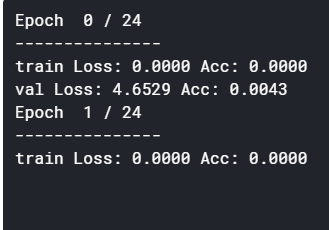I have also changed the optimizer to train only the final layer of efficeint net model .

# setting up the optimizer , loss func. & scheduler for training

``````from cutmix.utils import CutMixCrossEntropyLoss #for Stochastic Weight Averaging in PyTorch

from torchcontrib.optim import SWA

optimizer = SWA(base_optimizer, swa_start=5, swa_freq=5, swa_lr=0.05)

loss_fn = CutMixCrossEntropyLoss(True)

scheduler = torch.optim.lr_scheduler.StepLR(optimizer, step_size=7, gamma=0.1)
``````

Since the loss as well as the accuracy are both zero, I would recommend to debug the code step by step and check the data types as well as values of `loss`, `running_loss`, `running_correct`, `epoch_loss`, and `epoch_acc`. I guess that you might be using an integer division at some point, which would create the zeros.

PS: the usage of `.data` is not recommended, as it might have unwanted side effect.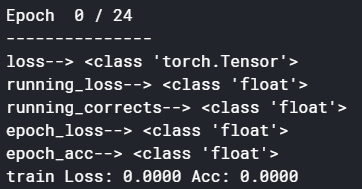I also typecast the values of dataset_sizes[phase] , float(dataset_sizes[phase] to float(dataset_sizes[phase]) , float(dataset_sizes[phase]) respectively as they were integer varibles before;

But the strange thing I noticed was here

# Iterate over data.

``````  for batch in dataloaders[phase]:
print(phase)
if torch.cuda.is_available():
inputs = batch['image'].cuda()
labels = batch['class'].cuda()
``````

it gave me this output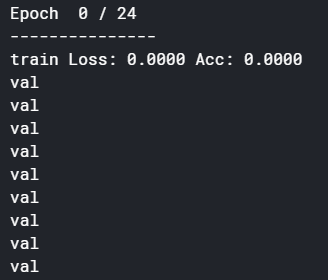means that phase is not going to train mode…am I right?

This might be still the case and as explained before, the indentation was the reason for it, which should have been fixed by now.